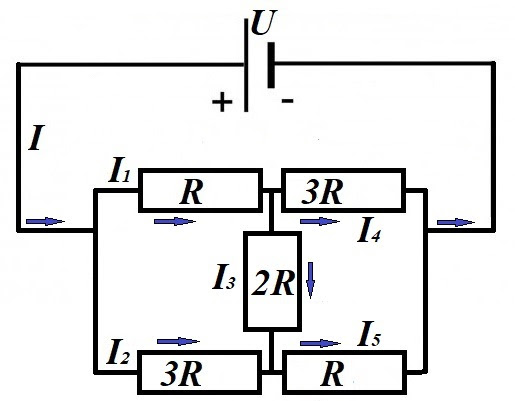## Saturday, April 11, 2020

### Unizor - Physics4Teens - Electromagnetism - DC Ohm's Law - Problems 3

Notes to a video lecture on http://www.unizor.com

Direct Current - Ohm's Law -
Problems 3

Problem A
Consider the following circuit with the voltage U and all resistances given.Ignore for now the arrows on the picture, they will be explained in the solution.
The task is to determine all the electric currents I1I2I3I4I5 going through each resistor and the current I in the common segment of the circuit.

Solution
This problem differs from seemingly analogous one presented before. There is no symmetry here, no points with the same electric potential that can be connected or disconnected for convenience.
We will solve it using the most general brute force approach.

First of all, we will randomly choose the direction of the unknown electric currents in each segment of a circuit. Randomly, because we don't really know the direction, except in the common segment with current I. In this segment the direction of current is from a positive terminal of the battery to a negative one (as you recall, by definition, it's opposite to the flow of electrons). In other cases we cannot determine the direction, and we choose any particular one. After the calculations it might be positive (if we correctly choose the direction) or negative (if in reality the direction is opposite).

We have six unknown values of the electric current in this problem. These values are related to each other because they have to obey certain laws of Physics, which we will use to have sufficient number of equations to find algebraically these unknown values.

Next, let's use the principle of balance of the current at every connection. Amount of electricity going into this connection per unit of time must be equal to amount electricity going out of it.
I = I1 + I2
I1 = I3 + I4
I2 + I3 = I5
I4 + I5 = I

To add more equations (we need six), let's consider a voltage drop on any part of a circuit with the same chosen direction of the current.

Going along the top of the circuit through resistors with resistances R and 3R, the combined voltage drop should be equal to a voltage between terminals of a battery
I1·R + I4·(3R) = U

Going along the bottom of the circuit through resistors with resistances 3R and R, the combined voltage drop should also be equal to a voltage between terminals of a battery
I2·(3R) + I5·R = U

Going along the top of the circuit through resistor with resistances R, then down through resistor 2R and right through resistor R, the combined voltage drop should also be equal to a voltage between terminals of a battery
I2·R + I3·(2R) + I5·R = U

Going along the bottom of the circuit through resistor with resistances 3R, then up through resistor 2R (against the current, so we should subtract the voltage drop) and right through resistor 3R, the combined voltage drop should also be equal to a voltage between terminals of a battery
I2·(3R) − I3·(2R) + I4·(3R) = U

We have got eight linear equations, with only six unknowns, which means that our system contains dependent equations, but it's OK, it can be algebraically solved.

Solution by substitution
(long by straight forward):

I=x,
I1=y,
I2=z,
I3=u,
I4=v,
I5=w,
A=U/R

x=y+z
y=u+v
z+u=w
v+w=x
y+3v=A
3z+w=A
y+2u+w=A
3z-2u+3v=A
______________
w=z+u
x=y+z
y=u+v
v+(z+u)=x
y+3v=A
3z+(z+u)=A
y+2u+(z+u)=A
3z-2u+3v=A
______________
x=y+z
y=u+v
v+z+u=x
y+3v=A
4z+u=A
y+3u+z=A
3z-2u+3v=A
______________
v=y-u
x=y+z
v+z+u=x
y+3v=A
4z+u=A
y+3u+z=A
3z-2u+3v=A
______________
x=y+z
(y-u)+z+u=x
y+3(y-u)=A
4z+u=A
y+3u+z=A
3z-2u+3(y-u)=A
______________
u=A-4z
x=y+z
y+z=x
same as above
y+3(y-u)=A
y+3u+z=A
3z-2u+3(y-u)=A
______________
x=y+z
4y-3(A-4z)=A
y+3(A-4z)+z=A
3z-5(A-4z)+3y=A
______________
z=x-y
4y+12z=4A
y-11z=-2A
3y+23z=6A
______________
3x-2y=A
-11x+12y=-2A
23x-20y=6A
______________
2y=3x-A
-11x+12y=-2A
23x-20y=6A
______________
-11x+6(3x-A)=-2A
23x-10(3x-A)=6A
______________
7x=4A
-7x=-4A
same as above
______________
x=4A/7
y=(12A/7 - A)/2 = 5A/14
z=3A/14 u=A-12A/14 = A/7
v=5A/14 - A/7 = 3A/14
w=3A/14 + A/7 = 5A/14
______________
I = 4U/(7R)
I1 = 5U/(14R)
I2 = 3U/(14R)
I3 = U/(7R)
I4 = 3U/(14R)
I5 = 5U/(14R)

Notice the anti-symmetry in resistors (top left is the same as bottom right R, top right is the same as bottom left 3R).
It results in equality of the corresponding currents:
I1 = I5,
I2 = I4.
______________

Knowing the current
I=4U/(7R),
we can calculate the total resistance of the circuit using the Ohm's Law:
Rcircuit = U/I = (7/4)·R